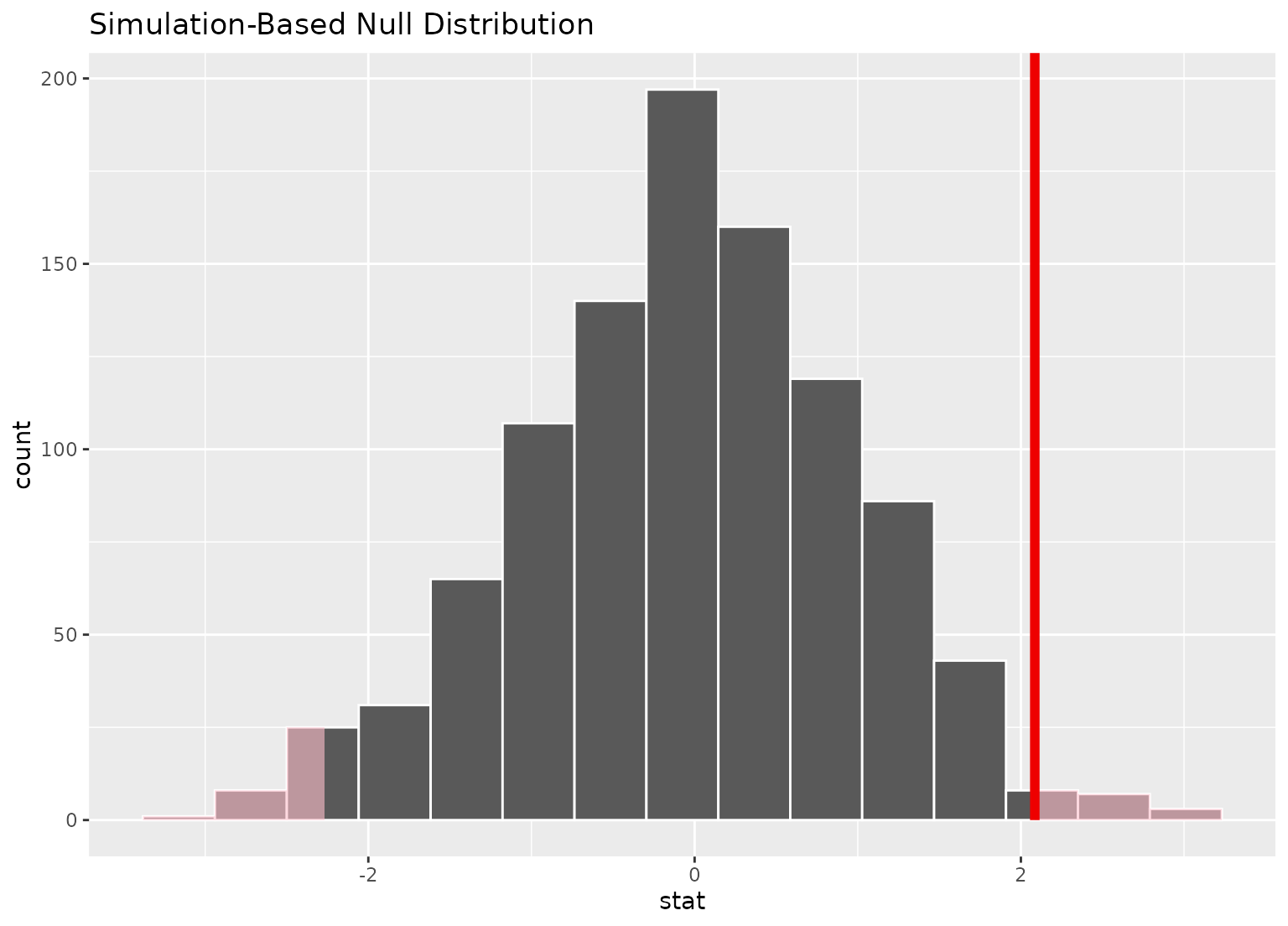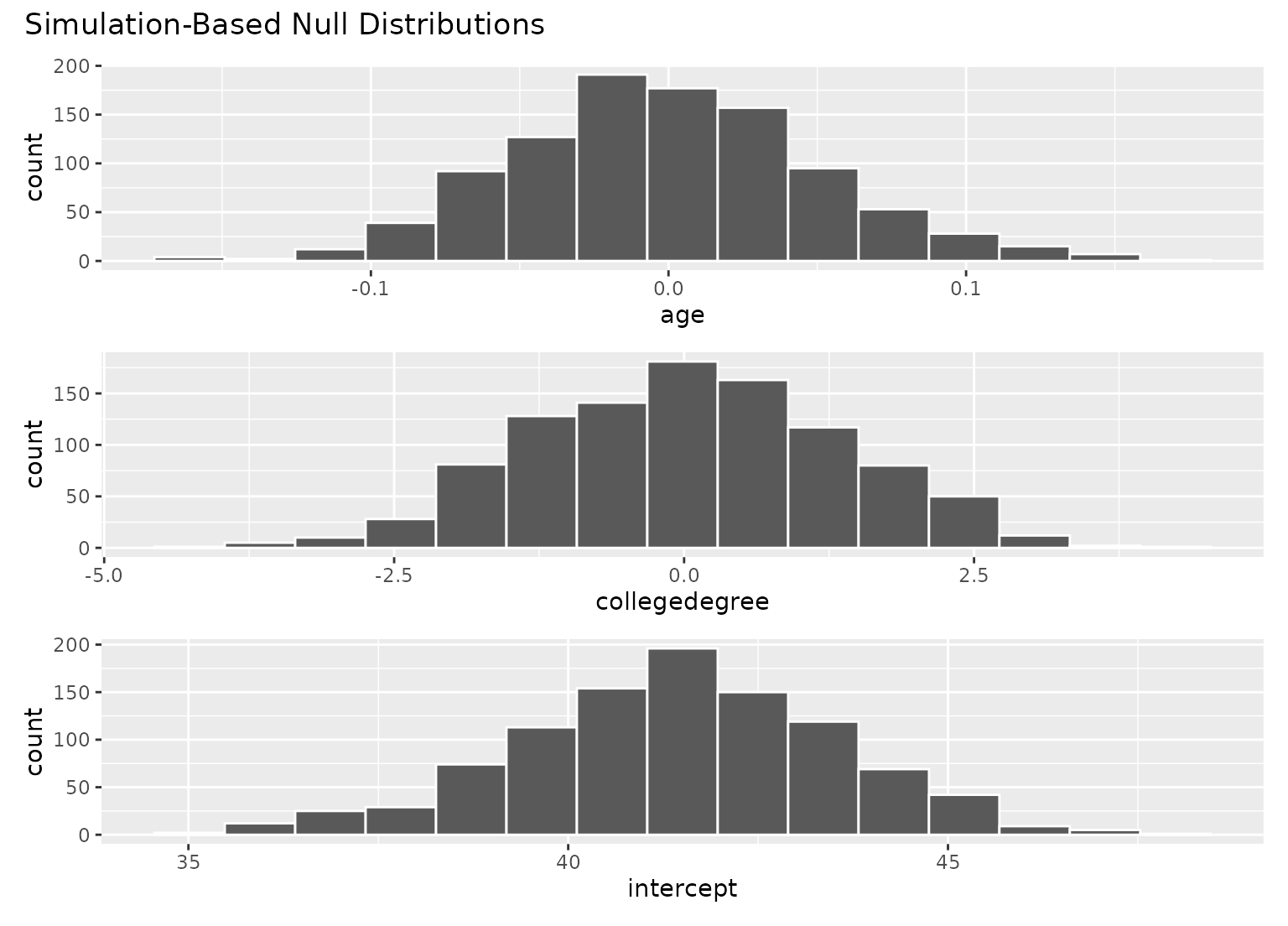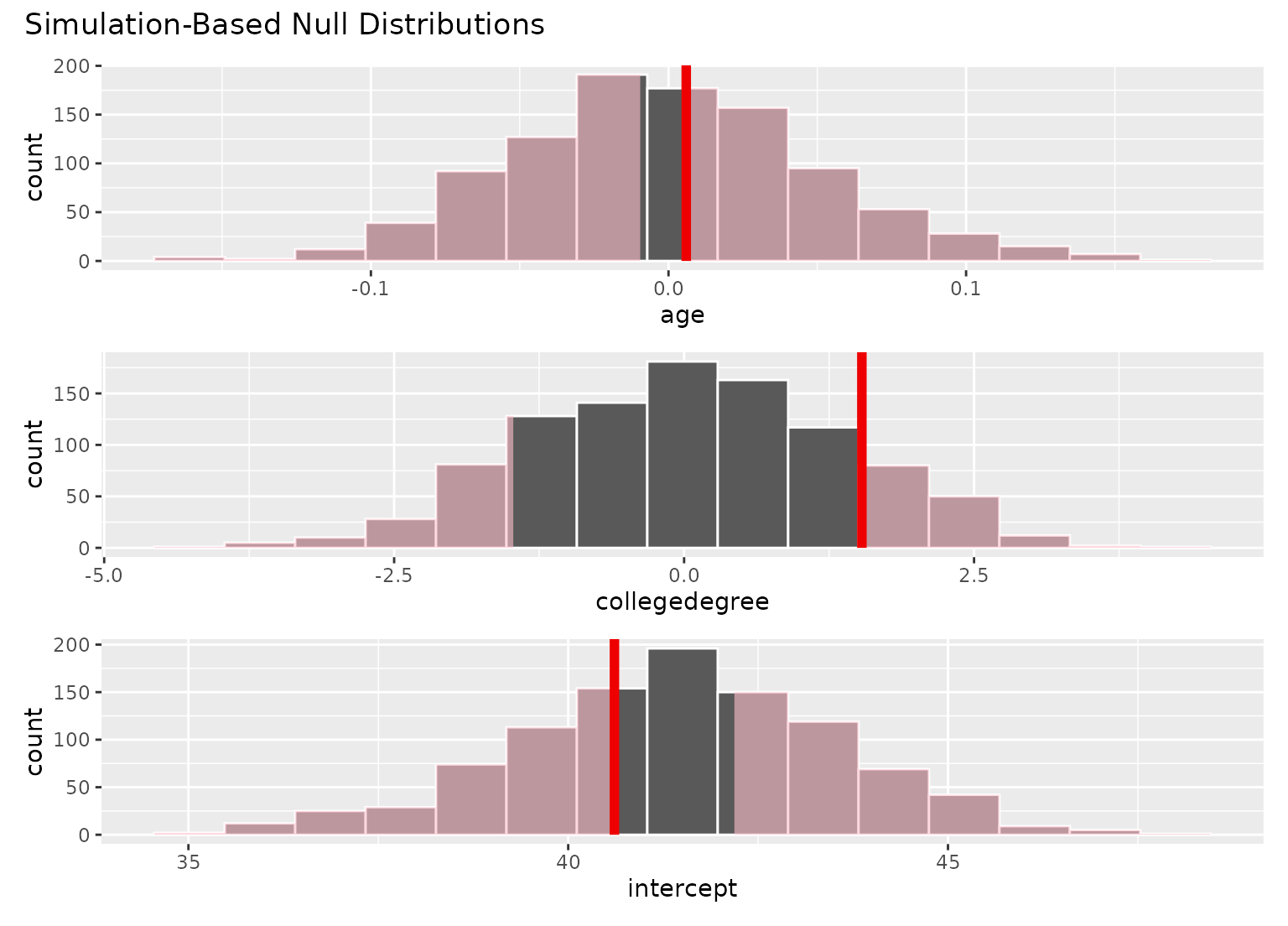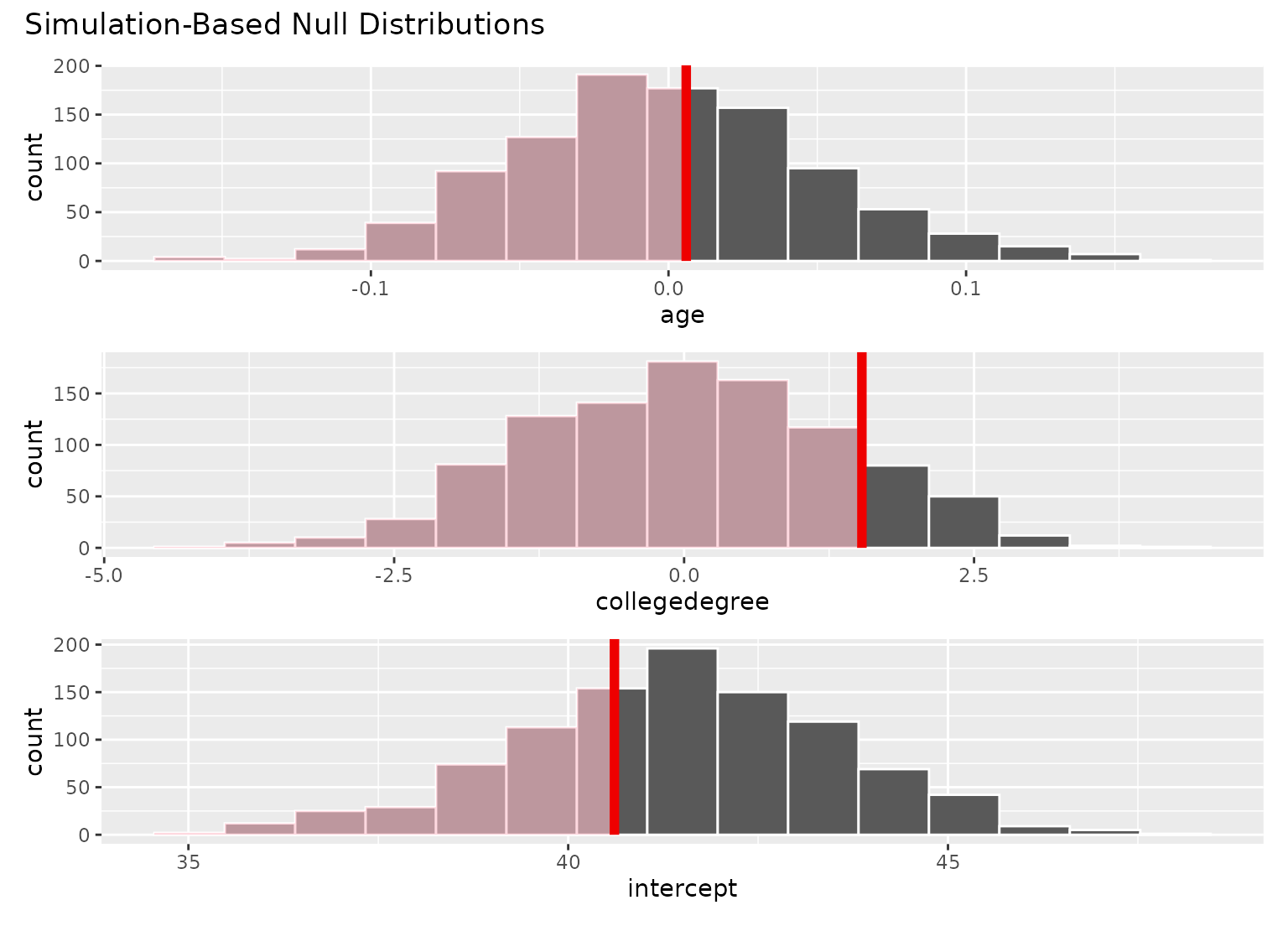shade_p_value() plots a p-value region on top of visualize() output. The output is a ggplot2 layer that can be added with +. The function has a shorter alias, shade_pvalue().

Learn more in vignette("infer").

shade_p_value(obs_stat, direction, color = "red2", fill = "pink", ...)

shade_pvalue(obs_stat, direction, color = "red2", fill = "pink", ...)

## Arguments

obs_stat The observed statistic or estimate. For calculate()-based workflows, this will be a 1-element numeric vector or a 1 x 1 data frame containing the observed statistic. For fit()-based workflows, a (p + 1) x 2 data frame with columns term and estimate giving the observed estimate for each term. A string specifying in which direction the shading should occur. Options are "less", "greater", or "two-sided". Can also give "left", "right", "both", "two_sided", "two sided", or "two.sided". If NULL, the function will not shade any area. A character or hex string specifying the color of the observed statistic as a vertical line on the plot. A character or hex string specifying the color to shade the p-value region. If NULL, the function will not shade any area. Other arguments passed along to \ggplot2\ functions. For expert use only.

## Value

If added to an existing infer visualization, a \ggplot2\ object displaying the supplied statistic on top of its corresponding distribution. Otherwise, an infer_layer list.

Other visualization functions: shade_confidence_interval()

## Examples

# find the point estimate---mean number of hours worked per week
point_estimate <- gss %>%
specify(response = hours) %>%
hypothesize(null = "point", mu = 40) %>%
calculate(stat = "t")

# ...and a null distribution
null_dist <- gss %>%
# ...we're interested in the number of hours worked per week
specify(response = hours) %>%
# hypothesizing that the mean is 40
hypothesize(null = "point", mu = 40) %>%
# generating data points for a null distribution
generate(reps = 1000, type = "bootstrap") %>%
# estimating the null distribution
calculate(stat = "t")

# shade the p-value of the point estimate
null_dist %>%
visualize() +
shade_p_value(obs_stat = point_estimate, direction = "two-sided")# you can shade confidence intervals on top of
# theoretical distributions, too!
null_dist_theory <- gss %>%
specify(response = hours) %>%
assume(distribution = "t")

null_dist_theory %>%
visualize() +
shade_p_value(obs_stat = point_estimate, direction = "two-sided")# \donttest{
# to visualize distributions of coefficients for multiple
# explanatory variables, use a fit()-based workflow

# fit 1000 linear models with the hours variable permuted
null_fits <- gss %>%
specify(hours ~ age + college) %>%
hypothesize(null = "independence") %>%
generate(reps = 1000, type = "permute") %>%
fit()

null_fits
#> # A tibble: 3,000 × 3
#> # Groups:   replicate [1,000]
#>    replicate term          estimate
#>        <int> <chr>            <dbl>
#>  1         1 intercept      43.1
#>  2         1 age            -0.0485
#>  3         1 collegedegree   0.562
#>  4         2 intercept      42.1
#>  5         2 age            -0.0180
#>  6         2 collegedegree   0.0745
#>  7         3 intercept      36.4
#>  8         3 age             0.121
#>  9         3 collegedegree   0.402
#> 10         4 intercept      42.6
#> # … with 2,990 more rows

# fit a linear model to the observed data
obs_fit <- gss %>%
specify(hours ~ age + college) %>%
fit()

obs_fit
#> # A tibble: 3 × 2
#>   term          estimate
#>   <chr>            <dbl>
#> 1 intercept     40.6
#> 2 age            0.00596
#> 3 collegedegree  1.53

# visualize distributions of coefficients
# generated under the null
visualize(null_fits)# fits with the observed fit for each term
visualize(null_fits) +# the direction argument will be applied
# to the plot for each term
visualize(null_fits) +